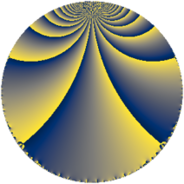# Properties

 Label 108.4.hLevel $108$ Weight $4$ Character orbit 108.h Rep. character $\chi_{108}(35,\cdot)$ Character field $\Q(\zeta_{6})$ Dimension $32$ Newform subspaces $2$ Sturm bound $72$ Trace bound $1$

# Learn more about

## Defining parameters

 Level: $$N$$ $$=$$ $$108 = 2^{2} \cdot 3^{3}$$ Weight: $$k$$ $$=$$ $$4$$ Character orbit: $$[\chi]$$ $$=$$ 108.h (of order $$6$$ and degree $$2$$) Character conductor: $$\operatorname{cond}(\chi)$$ $$=$$ $$36$$ Character field: $$\Q(\zeta_{6})$$ Newform subspaces: $$2$$ Sturm bound: $$72$$ Trace bound: $$1$$ Distinguishing $$T_p$$: $$5$$

## Dimensions

The following table gives the dimensions of various subspaces of $$M_{4}(108, [\chi])$$.

Total New Old
Modular forms 120 40 80
Cusp forms 96 32 64
Eisenstein series 24 8 16

## Trace form

 $$32q + 3q^{2} - q^{4} + 6q^{5} + O(q^{10})$$ $$32q + 3q^{2} - q^{4} + 6q^{5} - 20q^{10} - 2q^{13} + 78q^{14} - q^{16} + 234q^{20} + 15q^{22} + 198q^{25} - 132q^{28} - 78q^{29} - 687q^{32} - 191q^{34} - 8q^{37} - 891q^{38} + 34q^{40} + 156q^{41} + 48q^{46} + 194q^{49} + 1977q^{50} - 332q^{52} + 3006q^{56} - 314q^{58} - 2q^{61} + 410q^{64} - 690q^{65} - 4845q^{68} + 84q^{70} - 836q^{73} - 5874q^{74} - 495q^{76} - 978q^{77} + 682q^{82} + 248q^{85} + 8331q^{86} + 531q^{88} + 8724q^{92} + 168q^{94} + 16q^{97} + O(q^{100})$$

## Decomposition of $$S_{4}^{\mathrm{new}}(108, [\chi])$$ into newform subspaces

Label Dim. $$A$$ Field CM Traces $q$-expansion
$$a_2$$ $$a_3$$ $$a_5$$ $$a_7$$
108.4.h.a $$8$$ $$6.372$$ 8.0.$$\cdots$$.1 None $$3$$ $$0$$ $$-66$$ $$0$$ $$q+\beta _{7}q^{2}+(2\beta _{2}-2\beta _{3}-2\beta _{4}+\beta _{6}+\cdots)q^{4}+\cdots$$
108.4.h.b $$24$$ $$6.372$$ None $$0$$ $$0$$ $$72$$ $$0$$

## Decomposition of $$S_{4}^{\mathrm{old}}(108, [\chi])$$ into lower level spaces

$$S_{4}^{\mathrm{old}}(108, [\chi]) \cong$$ $$S_{4}^{\mathrm{new}}(36, [\chi])$$$$^{\oplus 2}$$